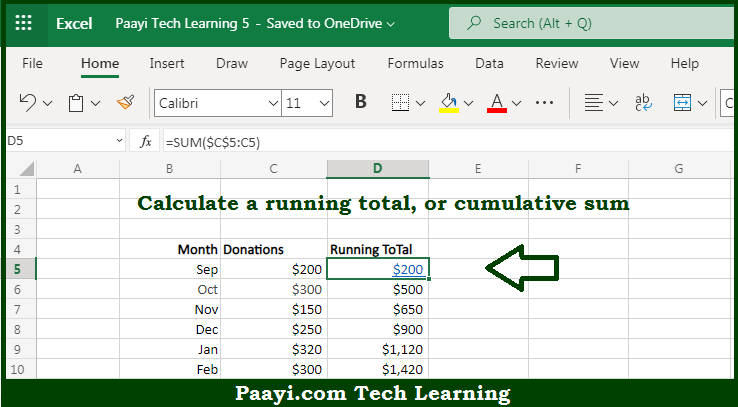# Learn How to SUM Running Total in Microsoft Excel

Written by | 0 Comments | 639 Views

In this article, you will learn how to COUNT various things in Microsoft Excel using a single/combination(s) of functions. You will also know how to SUM Running Total and see the generic formula.

SUM Running Total in Microsoft Excel

The main purpose of this formula is to calculate a running total or cumulative sum. Here we will learn how to sum the running total in Microsoft Excel. That implies, with the help of a formula based on the SUM function and mixed references you can able to calculate a running total or cumulative sum. So, with the help of this formula, you can able to sum the running total in Microsoft Excel.

General Formula to 3D SUMIF For Multiple Worksheets

=SUM(\$A\$1:A1)

The Explanation for the 3D SUMIF For Multiple WorksheetsSo we know that with the help of the given formula above you can able to calculate a running total or cumulative sum. Here we will learn how to sum the running total in Microsoft Excel. As we know that the above formula uses what is known as a "mixed reference" to create an "expanding range". A mixed reference is a reference that includes both absolute and relative parts. So, with the help of this formula, you can able to able to calculate a running total or cumulative sum.